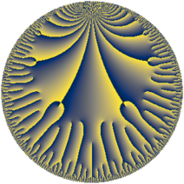# Properties

 Label 8.22.bLevel $8$ Weight $22$ Character orbit 8.b Rep. character $\chi_{8}(5,\cdot)$ Character field $\Q$ Dimension $20$ Newform subspaces $1$ Sturm bound $22$ Trace bound $0$

# Related objects

## Defining parameters

 Level: $$N$$ $$=$$ $$8 = 2^{3}$$ Weight: $$k$$ $$=$$ $$22$$ Character orbit: $$[\chi]$$ $$=$$ 8.b (of order $$2$$ and degree $$1$$) Character conductor: $$\operatorname{cond}(\chi)$$ $$=$$ $$8$$ Character field: $$\Q$$ Newform subspaces: $$1$$ Sturm bound: $$22$$ Trace bound: $$0$$

## Dimensions

The following table gives the dimensions of various subspaces of $$M_{22}(8, [\chi])$$.

Total New Old
Modular forms 22 22 0
Cusp forms 20 20 0
Eisenstein series 2 2 0

## Trace form

 $$20 q + 286 q^{2} + 409876 q^{4} + 118236748 q^{6} + 564950496 q^{7} + 3649699336 q^{8} - 62762119220 q^{9} + O(q^{10})$$ $$20 q + 286 q^{2} + 409876 q^{4} + 118236748 q^{6} + 564950496 q^{7} + 3649699336 q^{8} - 62762119220 q^{9} - 51269339528 q^{10} + 65316900136 q^{12} - 1924309104464 q^{14} - 2285680856096 q^{15} + 7793508571920 q^{16} + 1740714497000 q^{17} - 41325344023822 q^{18} - 78999934156016 q^{20} + 294957216952508 q^{22} + 335749622520224 q^{23} + 786737698607504 q^{24} - 1665114591247004 q^{25} - 2772669922448408 q^{26} - 4284340177681888 q^{28} + 3807691030523312 q^{30} - 8989263821171840 q^{31} - 9127378177656544 q^{32} + 5359113021386256 q^{33} + 19124803780998044 q^{34} + 39378114498954828 q^{36} - 41860137429498580 q^{38} + 121743910075345312 q^{39} - 160007944946664288 q^{40} - 84626460793452344 q^{41} + 178614544452770272 q^{42} - 27785097292304568 q^{44} + 184220935820050448 q^{46} + 149908985865280320 q^{47} + 1509829480382701344 q^{48} + 477689401234988532 q^{49} - 1722157324053237098 q^{50} - 1640429312330235856 q^{52} + 785391046636854392 q^{54} - 1936239868862269088 q^{55} - 3751496032099552448 q^{56} + 409355502574547024 q^{57} + 3182351472522780872 q^{58} + 2751731248604508192 q^{60} - 13336157978726113600 q^{62} - 8780950644120846560 q^{63} - 9021637553716933568 q^{64} + 6302857570713584320 q^{65} + 5291575090585638744 q^{66} + 40891724357029299816 q^{68} + 18077776609913587392 q^{70} + 96451291685825247456 q^{71} + 49841566955976579512 q^{72} - 21461290229157072184 q^{73} - 42953490504627871688 q^{74} - 206966483397015363224 q^{76} + 163869164384378609168 q^{78} - 284214874786393231680 q^{79} - 49904712513208125120 q^{80} + 1291808332257012164 q^{81} + 112698322843931711404 q^{82} + 178681601993395676224 q^{84} - 523293212085532715396 q^{86} + 517213147301817170208 q^{87} - 124028902608265335088 q^{88} + 17316387144455013640 q^{89} + 171098967229297148360 q^{90} + 827091233447044225632 q^{92} - 165060487582514765280 q^{94} - 2333725338330109674784 q^{95} - 715448174391177540544 q^{96} + 9560220979460741928 q^{97} + 137273120368488603630 q^{98} + O(q^{100})$$

## Decomposition of $$S_{22}^{\mathrm{new}}(8, [\chi])$$ into newform subspaces

Label Dim $A$ Field CM Traces $q$-expansion
$a_{2}$ $a_{3}$ $a_{5}$ $a_{7}$
8.22.b.a $20$ $22.358$ $$\mathbb{Q}[x]/(x^{20} - \cdots)$$ None $$286$$ $$0$$ $$0$$ $$564950496$$ $$q+(14-\beta _{1})q^{2}+(1+3\beta _{1}-\beta _{2})q^{3}+\cdots$$